# Conditional Formatting Based on a Formula Part IExcel’s conditional formatting capability allows the user to format cells based on the contents of a cell. In our June-August 2017 newsletters, we discussed the built-in conditional formatting capabilities of Excel (Top Bottom Rules, Highlight Cells, Data Bars, Color Scales, and Icon Sets.) In the next few newsletters, we will show how you can use Excel formulas to create complex formatting rules.

To utilize Excel’s Conditional Formatting with a formula option we proceed as follows:

1. Select the range where the formatting will be applied.
2. After selecting Conditional Formatting from the Home Tab select New Rule.
3. Choose Use a Formula.
4. With the cell in the upper left-hand corner of selected range type in a formula starting with =.
5. This formula will copy across and down-selected range (watch your \$ signs!) Whenever the copied formula is True, the desired format will be used.

In the file Beckerfeb2020.xlsx we illustrate the use of the Conditional Formatting with a Formula capability. Column E of the worksheet Amazon (see Figure 1) contains quarterly revenues for Amazon.com (in millions of dollars.) When revenue increased from the previous quarter, we want to highlight the revenue in green and when revenue decreased, we want to highlight the revenue in red.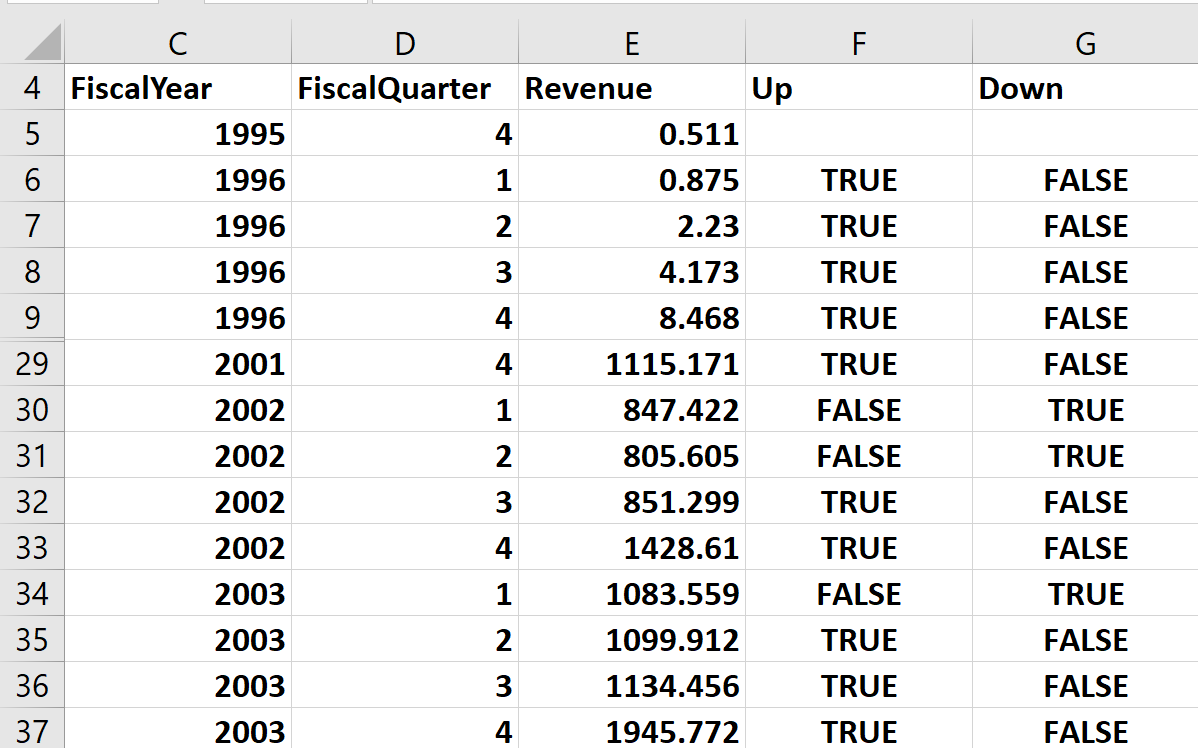Figure 1 Amazon.com Data.

To begin, we select the cell range E6:E85 where we want to apply the format. Then from the Home tab, we choose Conditional Formatting and choose New Rule Use a Formula. Now think about what must be true for E6 to be formatted in green. We need E6>E5. If this formula is copied down then whenever the formula is True (see Column F), we should format the quarter’s revenue in green. Therefore, we fill in the Select a Rule Type dialog box as shown in Figure 2. After clicking OK, all revenues that were larger than the previous quarter are highlighted in green.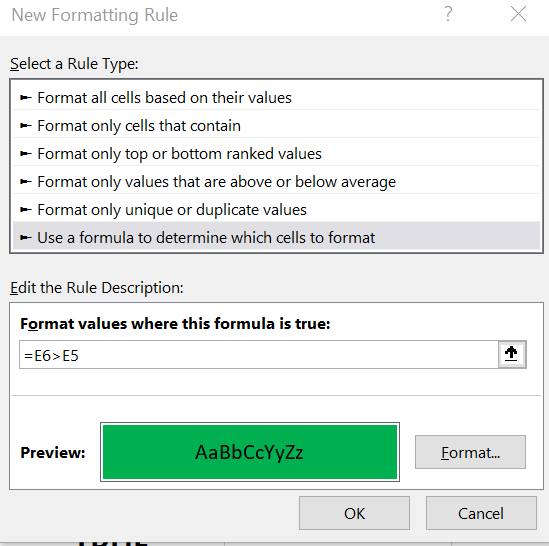Figure 2 Creating the Formula to format Revenues in Green

We want to format cell E6 in red if E6<E5. If this formula is copied down then whenever the formula is True (see Column G, we should format the quarter’s revenue in green. Therefore, we fill in the Select a Rule Type dialog box as shown in Figure 3. After clicking OK, all revenues that were smaller than the previous quarter are highlighted in red.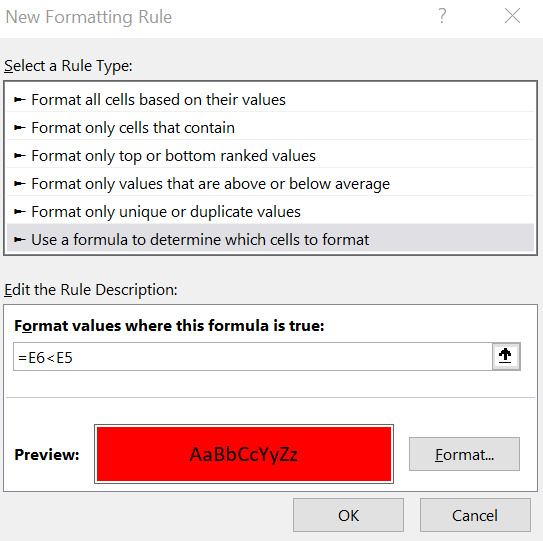Figure 3 Creating the Formula to Format Revenues in Red

As shown in the worksheet Format (see Figure 4) the revenues that were larger than the previous quarter are highlighted in green and the revenues that were smaller than the previous quarter are highlighted in red.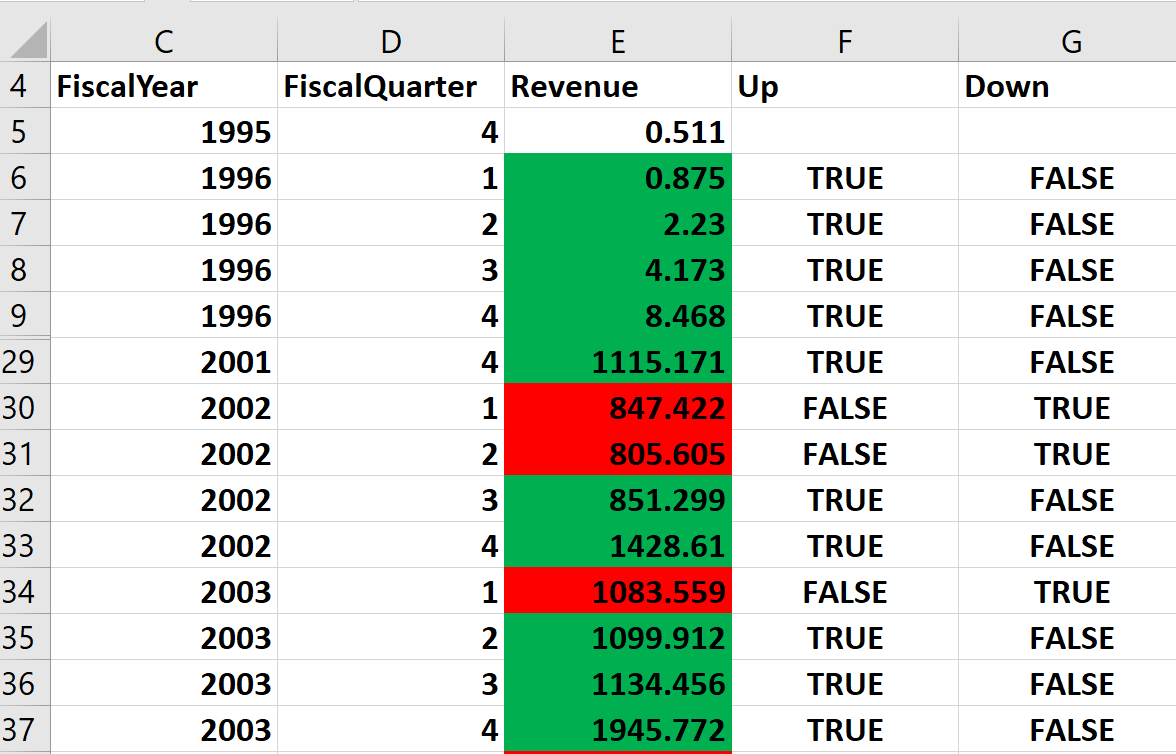Figure 4 Revenue increases are highlighted in Green and Revenue decreases are highlighted in Red.

Note that Column E is highlighted in Green if and only if Column F contains TRUE Green and Column E is highlighted in Red if and only if Column G contains TRUE.

If your cursor is anywhere in the range (E6:E85) where the format was applied, selecting Manage Rules from the Conditional Formatting menu will show you the conditional formats (see Figure 5) applied to that range.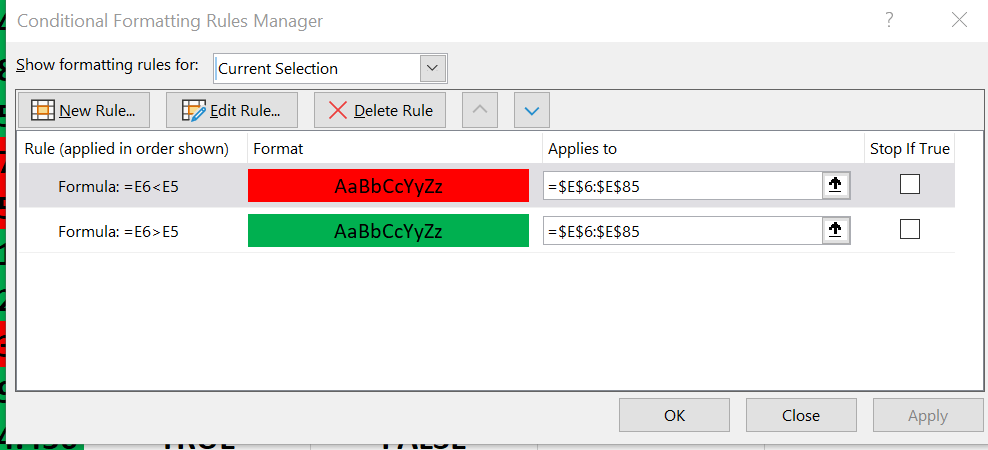Figure 5 Manage Rules shows the Conditional Formats applied to the Range E6:E85

Next month, we will show you more complex examples that further illustrate the amazing capabilities of the Conditional Formatting with a Formula option.